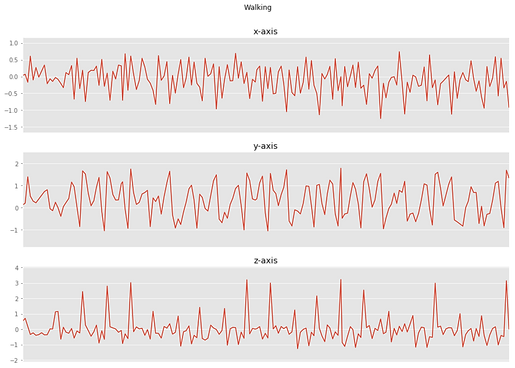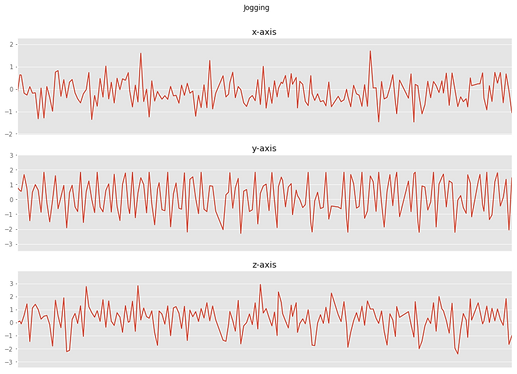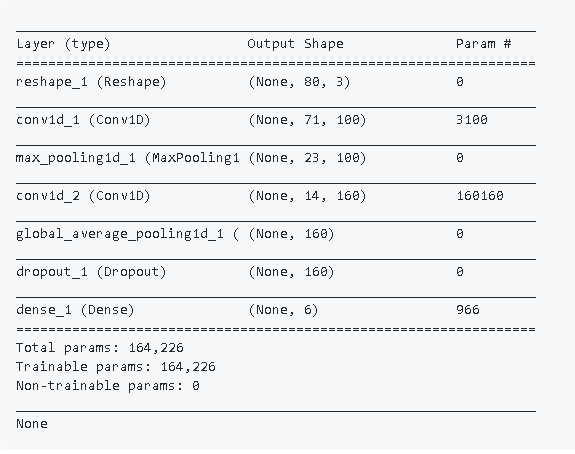### Human Activity Recognition using CNN on Inertial Data

This project aimed to develop a Convolution Network to perform recognition of physical activities using on-body Inertial Measurement Sensors. Tensorflow and Keras APIs were used for the development of a 1D Sequential CNN of 7 Layers.

An old version of the Actitracker dataset from the Wireless Sensor Data Mining (WISDM) lab at Fordham University, NY, USA was used to train and test the network. The latest dataset can be downloaded from:

The activity states provided in the dataset are jogging, walking, ascending stairs, descending stairs, sitting and standing. The data was collected from 36 users using a smartphone in their pocket with the 20Hz sampling rate (20 values per second).

### Pipeline

The training process starts by reading the data and normalizing it. This normalized data is then segmented into time slices of window size 80 which translates to 4 seconds long chunks of data. These chunks are then randomly split into training and test sets. For the results shown in this report, 70% data as taken into the test set and the remaining was used in the test set for the validation of the training algorithm. This training data which was fed to a 1D CNN network is described below.

The one window size long plots for each class of the normalized data are shown belowUpstairs

Walking

I'm a paragraph. Click here to add your own text and edit me. It's easy.Downstairs

StandingSitting

Jogging

### CNN Network

A 1D CNN network was used considering the dimensions of the data. Each row of the data consists of the x,y,z accelerations from the accelerometer and the height of the layer determines the number of instances of data equalling the window size which is 80 in our case. Only the size of the input and output layers needs to be specified explicitly. The network estimates the size of the hidden layers on its own.

The network used here is of a sequential type which means that it's basically a stack of layers. These layers include:

• Input layer

• First 1D CNN Layer

• A max pooling layer

• Second 1D CNN Layer

• An average pooling layer

• A dropout layer

• A fully connected Softmax Activated layer

Input Layer: The input data consists of 80-time slices long instances of 3-axis accelerometer. Hence, the size of the input layer needs to be reshaped to 80x3. The data passes through the input layer as a vector of length 240. The output for this layer is 80x3.

First 1D CNN Layer: This defines a filter of kernel size 10. 100 such filters are defined in this layer to enable it to learn 100 different features. The output for this layer is a 71x100 matrix of neurons where the weights of each filter are defined column-wise.

A max pooling layer: This is used to reduce the complexity of the output and to prevent overfitting of the data. Using a pooling layer size of 3 reduces the size of the output matrix to 1/3rd of the input matrix.

Second 1D CNN Layer: This layer enables the network to pick up higher level features which were missed in the First CNN layer. The output of this layer is a 14x160 matrix.

Average pooling layer: This averages the value of two weights in the network thereby further reducing overfitting. The output is 1x160 matrix of neurons.

Dropout layer: This randomly assigns a weight of 0 to the neurons in the network. A value of 0.5 indicates that 50% of the neurons turn 0.

Fully connected Softmax Activated layer: This reduces the output to the desired height of 6 which indicates the number of activity classes in the data. Softmax forces all six outputs of the neural network to sum up to one.

Results

The network was successfully trained to recognize human activities using data obtained from on-body 3-axis accelerometers.

Test accuracy of 92.66 % and training accuracy of 93.73% was achieved. The algorithm converges in 11 epochs equalling approximately 46 seconds.

### Dataset

Actitracker dataset from the Wireless Sensor Data Mining (WISDM) lab at Fordham University, NY, USA was used to train and test the network. The latest dataset can be downloaded from: